NCERT Solutions for Class 9 Maths Chapter 5 Triangles Ex 5.4 are part of NCERT Solutions for Class 9 Maths. Here we have given NCERT Solutions for Class 9 Maths Chapter 5 Triangles Ex 5.4.

 Board CBSE Textbook NCERT Class Class 9 Subject Maths Chapter Chapter 5 Chapter Name Triangles Exercise Ex 5.4 Number of Questions Solved 6 Category NCERT Solutions

## NCERT Solutions for Class 9 Maths Chapter 5 Triangles Ex 5.4

Question 1.
Show that in a right angled triangle, the hypotenuse is the longest side.
Solution:
Let ABC be a right angled triangle, such that ∠ ABC = 90°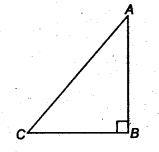We know that,
∠ABC + ∠BCA + ∠CAB = 180° (By A property)
⇒ 90° + ∠BCA + ∠CAB = 180°
⇒ ∠BCA + ∠CAB = 90°
From above, we have ∠ BCA and ∠ CAB are acute angles.
⇒ ∠BCA < 90°
and ∠CAB < 90°
⇒ ∠BCA < ∠ABC
and ∠CAB < ∠ABC
⇒ AB < AC and BC < AC (∵ Side opposite to greater angle is longer)
Hence, the hypotenuse (AC) is the longest side.

Question 2.
In figure, sides AB and AC of ∆ABC are extended to points P and Q respectively. Also, ∠PBC < ∠QCB. Show that AC > AB.
Solution:
We know that,∠ACB + ∠QCB = 180° (Linear pair)…(i)
and ∠ABC+ ∠PBC = 180° (Linear pair)…(ii)
From Eqs. (i) and (ii), we have
∠ABC + ∠PBC = ∠ACB + ∠QCB ….(iii)
But ∠PBC < ∠QCB (Given)…(iv) From Eqs. (iii) and (iv), we have ∠ ABC > ∠ ACB
⇒ AC > AB
(∵ Side opposite to greater angle is longer)

Question 3.
In figure, ∠B <∠ A and ∠C <∠ D. Show that AD < BC.
Solution:Question 4.
AB and CD are respectively the smallest and longest sides of a quadrilateral ABCD (see figure). Show that ∠ A> ∠C and ∠B > ∠D.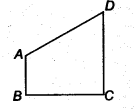Solution:
Given ABCD is a quadrilateral. AB is the smallest side and CD is the longest side.
To prove ∠A > ∠C and ∠B > ∠D
Construction Join A to C and B to D.
Proof In ∆ABC, we have AB is the smallest side.
∴ AB < BC
⇒ ∠5 < ∠1(∵ Angle opposite to longer side is greater) …(i)
In ∆ADC, we have CD is the largest side.Question 5.
In figure, PR > PQ and PS bisect ∠QPR. Prove that
∠PSR >∠PSQ.Solution:
In ∆ PQR, we have
PR > PQ (Given)
⇒ ∠ PQR > ∠ PRQ …(i)
(∵ Angle opposite to longer side is greater)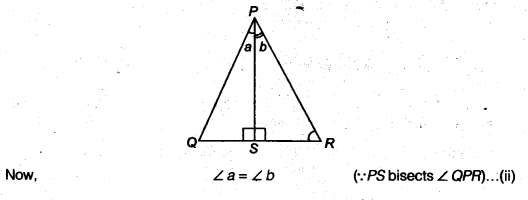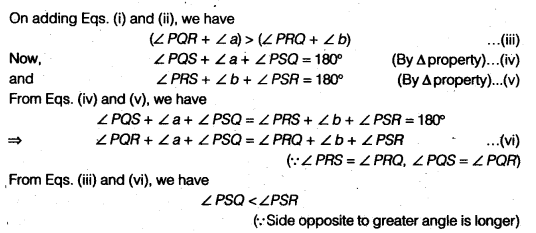Question 6.
Show that of all line segments drawn from a given point not on it, the perpendicular line segment is the shortest.
Solution:
Given x is a line and A is a point not lying on x. AB ⊥ x, Cis any point on x other than B.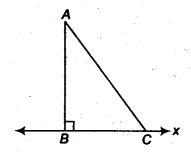To prove AB∠C
⇒ AC> AB
(∵ the Side opposite to greater angle is longer)
⇒ AB < AC
Hence, the perpendicular line segment is the shortest.
We hope the NCERT Solutions for Class 9 Maths Chapter 5 Triangles Ex 5.4 help you. If you have any query regarding NCERT Solutions for Class 9 Maths Chapter 5 Triangles Ex 5.4, drop a comment below and we will get back to you at the earliest.